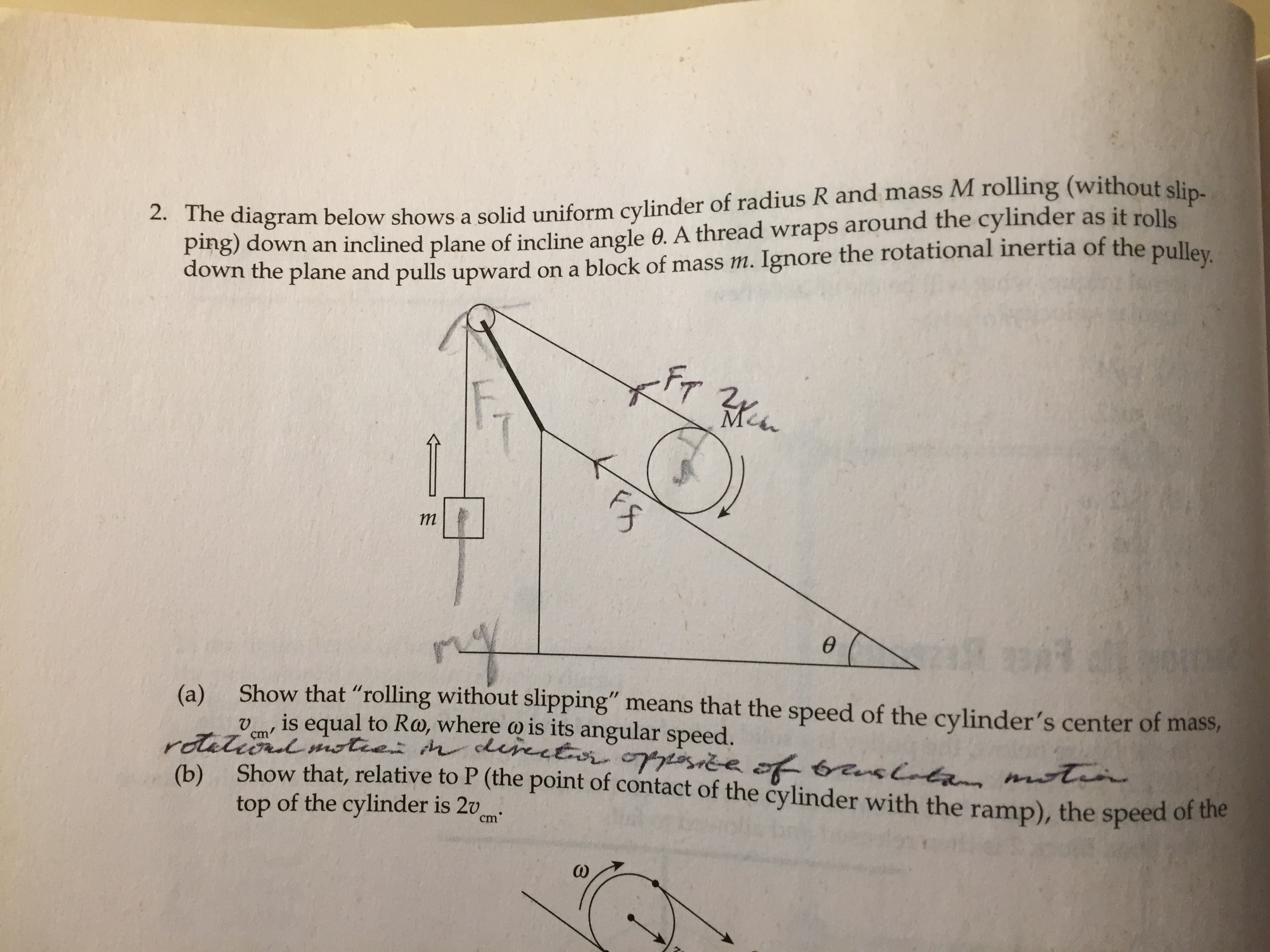# A mechanics problem

Originally published at 狗和留美者不得入内. You can comment here or there.

Today, I solved one from the Cracking the AP Physics C Exam 2008 Edition, which I had bought in high school. It isQuestions (c), (d), and (e) are not included. They are to determine the relationship between the magnitude of the acceleration of the block and the linear acceleration of the cylinder, to determine the acceleration of the cylinder, and to determine the acceleration of the block respectively. It is obvious that from (b) that the magnitude of the acceleration of the block is twice the linear acceleration of the cylinder, and the answer to (e) can be derived from that of (d) via this. As for (d), I first solved it with a free body diagram and Newton’s laws. Then, my ultra gifted friend Brian Bi suggested using the Euler-Lagrange equations, which simplifies things greatly, to the extent that he characterized himself as having forgotten how to solve mechanics problems the way I had done (obviously not true, but you get what he’s saying). I was able to work it out this way shortly, getting the same answer.Note: I left overlooked the$4$ coefficient initially and only filled it in at the end. It is still omitted in some of the intermediate equations.

Tags:

### Recent Posts from This Journal

• #### How weak convergence and weak topology arises naturally from infinite dimensional vector spaces

Originally published at 狗和留美者不得入内. You can comment here or there. Let be a vector space over endowed with an inner product . Assume that…

• #### Using the Minkowski functional to prove separation of sets via a hyperplane

Originally published at 狗和留美者不得入内. You can comment here or there. We shall use n.v.s to refer to normed vector space. Definition 1 Let be a…

• #### On the Baire category theorem and the open mapping theorem

Originally published at 狗和留美者不得入内. You can comment here or there. Theorem 1 (Baire Category Theorem) Let be a complete metric space. The…

• Post a new comment

#### Error

Anonymous comments are disabled in this journal

default userpic

Your reply will be screened

• 0 comments